## GRIDS

This chapter covers how to determine and report positions on the ground in terms of their locations on a map. Knowing where you are (position fixing) and being able to communicate that knowledge is crucial to successful land navigation as well as to the effective employment of direct and indirect fire, tactical air support, and medical evacuation. It is essential for valid target acquisition; accurate reporting of NBC contamination and various danger areas; and obtaining emergency resupply. Few factors contribute as much to the survivability of troops and equipment and to the successful accomplishment of a mission as always knowing where you are. The chapter includes explanations of geographical coordinates, Universal Transverse Mercator grids, the military grid reference system, and the use of grid coordinates.

### 4-1. REFERENCE SYSTEM

In a city, it is quite simple to find a location; the streets are named and the buildings have numbers. The only thing needed is the address. However, finding locations in undeveloped areas or in unfamiliar parts of the world can be a problem. To cope with this problem, a uniform and precise system of referencing has been developed.

### 4-2. GEOGRAPHIC COORDINATES

One of the oldest systematic methods of location is based upon the geographic coordinate system. By drawing a set of east-west rings around the globe (parallel to the equator), and a set of north-south rings crossing the equator at right angles and converging at the poles, a network of reference lines is formed from which any point on the earth’s surface can be located.

a.   The distance of a point north or south of the equator is known as its latitude. The rings around the earth parallel to the equator are called parallels of latitude or simply parallels. Lines of latitude run east-west but north-south distances are measured between them.

b.   A second set of rings around the globe at right angles to lines of latitude and passing through the poles is known as meridians of longitude or simply meridians. One meridian is designated as the prime meridian. The prime meridian of the system we use runs through Greenwich, England and is known as the Greenwich meridian. The distance east or west of a prime meridian to a point is known as its longitude. Lines of longitude (meridians) run north-south but east-west distances are measured between them (Figures 4-1 and 4-2).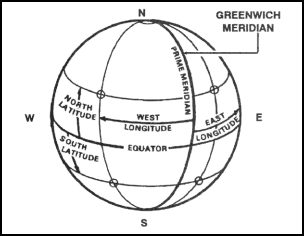Figure 4-1. Prime meridian and equator.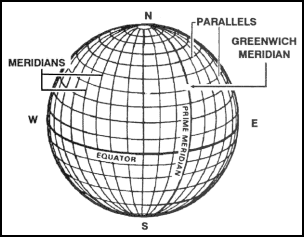Figure 4-2. Reference lines.

c.   Geographic coordinates are expressed in angular measurement. Each circle is divided into 360 degrees, each degree into 60 minutes, and each minute into 60 seconds. The degree is symbolized by ° , the minute by ′, and the second by ″. Starting with 0° at the equator, the parallels of latitude are numbered to 90° both north and south. The extremities are the north pole at 90° north latitude and the south pole at 90° south latitude. Latitude can have the same numerical value north or south of the equator, so the direction N or S must always be given. Starting with 0° at the prime meridian, longitude is measured both east and west around the world. Lines east of the prime meridian are numbered to 180° and identified as east longitude; lines west of the prime meridian are numbered to 180° and identified as west longitude. The direction E or W must always be given. The line directly opposite the prime meridian, 180° , may be referred to as either east or west longitude. The values of geographic coordinates, being in units of angular measure, will mean more if they are compared with units of measure with which we are more familiar. At any point on the earth, the ground distance covered by one degree of latitude is about 111 kilometers (69 miles); one second is equal to about 30 meters (100 feet). The ground distance covered by one degree of longitude at the equator is also about 111 kilometers, but decreases as one moves north or south, until it becomes zero at the poles. For example, one second of longitude represents about 30 meters (100 feet) at the equator; but at the latitude of Washington, DC, one second of longitude is about 24 meters (78 feet). Latitude and longitude are illustrated in Figure 4-3.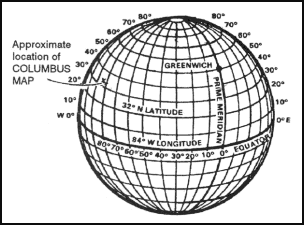Figure 4-3. Latitude and longitude.

d.   Geographic coordinates appear on all standard military maps; on some they may be the only method of locating and referencing a specific point. The four lines that enclose the body of the map (neatlines) are latitude and longitude lines. Their values are given in degrees and minutes at each of the four corners. On a portion of the Columbus map (Figure 4-4), the figures 32° 15’ and 84° 45’ appear at the lower right corner. The bottom line of this map is latitude 32° 15’00″N, and the line running up the right side is longitude 84° 45’00”W. In addition to the latitude and longitude given for the four corners, there are, at regularly spaced intervals along the sides of the map, small tick marks extending into the body of the map. Each of these tick marks is identified by its latitude or longitude value. Near the top of the right side of the map is a tick mark and the number 20’. The full value for this tick marks is 32° 20’00” of latitude. At one-third and two-thirds of the distance across the map from the 20’ tick mark will be found a cross tick mark (grid squares 0379 and 9679) and at the far side another 20’ tick mark. By connecting the tick marks and crosses with straight lines, a 32° 20’00” line of latitude can be added to the map. This procedure is also used to locate the 32° 25’00” line of latitude. For lines of longitude, the same procedure is followed using the tick marks along the top and bottom edges of the map.

e.   After the parallels and meridians have been drawn, the geographic interval (angular distance between two adjacent lines) must be determined. Examination of the values given at the tick marks gives the interval. For most maps of scale 1:25,000, the interval is 2’30”. For the Columbus map and most maps of scale 1:50,000, it is 5’00”. The geographic coordinates of a point are found by dividing the sides of the geographic square in which the point is located into the required number of equal parts. If the geographic interval is 5’00” and the location of a point is required to the nearest second, each side of the geographic square must be divided into 300 equal parts (5’00” = 300”), each of which would have a value of one second. Any scale or ruler that has 300 equal divisions and is as long as or longer than the spacing between the lines may be used.

f.   The following steps will determine the geographic coordinates of Wilkinson Cemetery (northwest of the town of Cusseta) on the Columbus map.

(1)   Draw the parallels and meridians on the map that encloses the area around the cemetery.

(2)   Determine the values of the parallels and meridians where the point falls.

Latitude 32° 15’00” and 32° 20’00”.

Longitude 84° 45’00” and 84° 50’00”.

(3)   Determine the geographic interval (5’00” = 300”).

(4)   Select a scale that has 300 small divisions or multiples thereof (300 divisions, one second each; 150 divisions, two seconds each; 75 divisions, four seconds each, and so forth).

(5)   To determine the latitude—

(a)   Place the scale with the 0 of the scale on the latitude of the lowest number value (32° 15’00”) and the 300 of the scale on the highest numbered line (32° 20’00”) (1, Figure 4-4).

(b)   While keeping the 0 and 300 on the two lines, slide the scale (2, Figure 4-4) along the parallels until the Wilkinson Cemetery symbol is along the edge of the numbered scale.

(c)   Read the number of seconds from the scale (3, Figure 4-4), about 246.

(d)   Convert the number of seconds to minutes and seconds (246” = 4’06”) and add to the value of the lower numbered line (32° 15’00” + 4’06” = 32° 19’06”) (4, Figure 4-4).

RESULTS:

• The latitude is 32° 19’06”, but this is not enough.

• The latitude 32° 19’06” could be either north or south of the equator, so the letter N or S must be added to the latitude. To determine whether it is N or S, look at the latitude values at the edge of the map and find the direction in which they become larger. If they are larger going north, use N; if they are larger going south, use S.

• The latitude for the cemetery is 32° 19’06”N.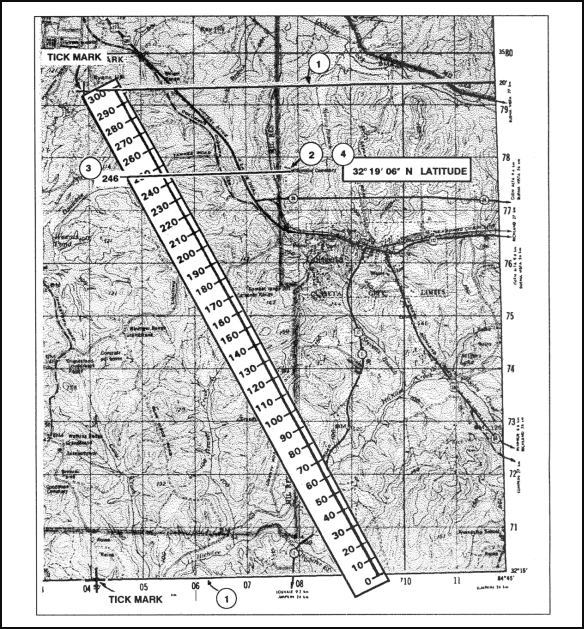Figure 4-4. Determining latitude.

(6)   Determine the longitude, repeat the same steps but measure between lines of longitude and use E and W. The geographic coordinates of Wilkinson Cemetery should be about 32° 19’06”N and 84° 47’32”W (Figure 4-5).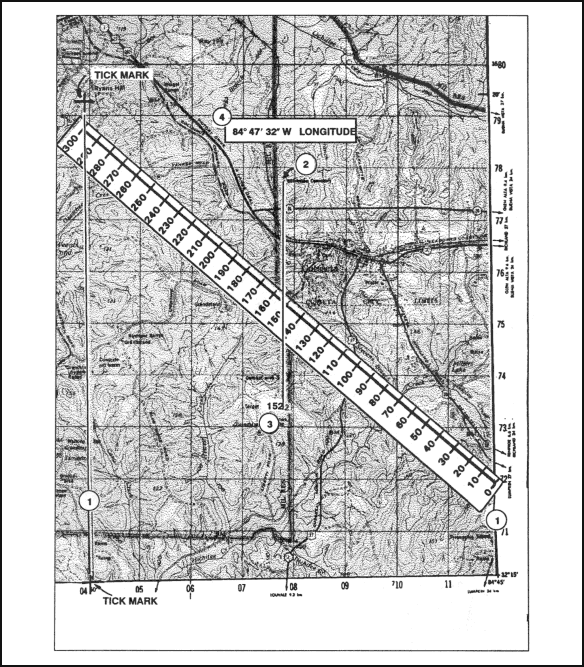Figure 4-5. Determining longitude.

g.   To locate a point on the Columbus map (Figure 4-6) when knowing the geographic coordinates, many of the same steps are followed. To locate 32° 25’28”N and 84° 50’56”W, first find the geographic lines within which the point falls: latitude 32° 25’00” and 32° 30’0”; and longitude 84° 50’00” and 84° 55’00”. Subtract the lower latitude/longitude from the higher latitude/longitude.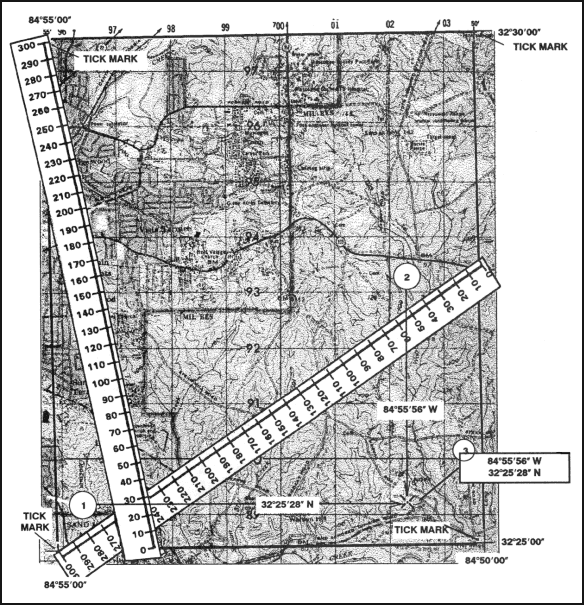Figure 4-6. Determining geographic coordinates.

(1)   Place the 0 of the scale on the 32° 25’00” line and the 300 on the 32° 30’00”. Make a mark at the number 28 on the scale (the difference between the lower and higher latitude).

(2)   Place the 0 of the scale on the 84° 50′00″ line and the 300 on the 84° 50′55″. Make a mark at the number 56 on the scale (the difference between the lower and higher longitude.

(3)   Draw a vertical line from the mark at 56 and a horizontal line from the mark at 28; they intersect at 32 25’28”N and 84 50’56”W.

h.   If you do not have a scale or ruler with 300 equal divisions or a map whose interval is other than 5’00”, use the proportional parts method. Following the steps determines the geographic coordinates of horizontal control station 141.

(1)   Locate horizontal control station 141 in grid square (GL0784) (Figure 4-7).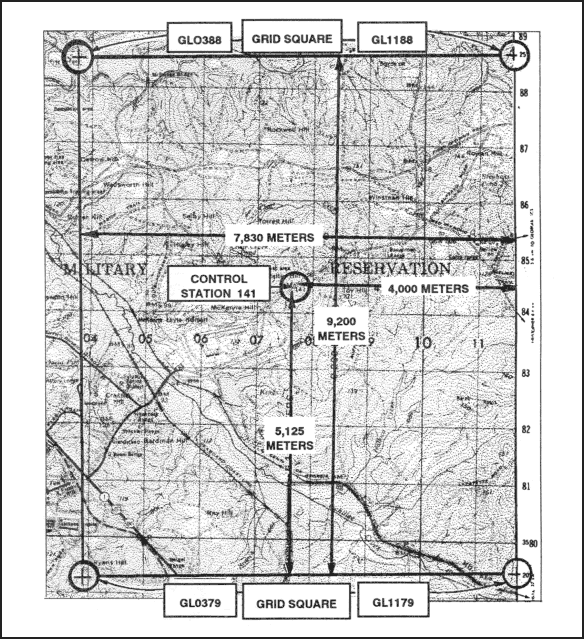Figure 4-7. Using the proportional parts method.

(2)   Find a cross in grid square GL0388 and a tick mark in grid square GL1188 with 25’.

(3)   Find another cross in grid square GL0379 and a tick mark in grid square GL1179 with 20’.

(4)   Enclose the control station by connecting the crosses and tick marks. The control station is between 20’ and 25’ (Figure 4-7).

(5)   With a boxwood scale, measure the distance from the bottom line to the top line that encloses the area around the control station on the map (total distance) (Figure 4-7).

(6)   Measure the partial distance from the bottom line to the center of the control station (Figure 4-7). These straight-line distances are in direct proportion to the minutes and seconds of latitude and are used to set up a ratio.

RESULTS:

• The total distance is 9,200 meters, and the partial distance is 5,125 meters (Figure 4-7).

• With the two distances and the five-minute interval converted to seconds (300”), determine the minutes and seconds of latitude using the following formula:

• 5,125 x 300 = 1,537,500

• 1,537,500 ÷ 9,200 = 167

• 167 ÷ 60 = 2’47”

• Add 2’47” to 32° 20’00” = 32° 20’47”

(7)   Follow the same procedures to determine minutes and seconds of longitude (Figure 4-7).

RESULTS:

• The total distance is 7,830 meters, and the partial distance is 4,000 meters (Figure 4-7).

• 4,000 x 300 = 1,200,000

• 1,200,000 ÷ 7,830 = 153

• 153 ÷ 60 = 2’33”

• Add 2’33” to 84° 45’ = 84° 47’33”N

(8)   The geographic coordinates of horizontal control station 141 in grid square GL0784 are 32° 22’47”N latitude and 84° 47’33”W longitude.

NOTE:

i.   The maps made by some nations do not have their longitude values based on the prime meridian that passes through Greenwich, England. Table 4-1 shows the prime meridians that may be used by other nations. When these maps are issued to our soldiers, a note usually appears in the marginal information giving the difference between our prime meridian and the one used on the map.

Amsterdam, Netherlands

4° 53’01”E

Athens, Greece

23° 42’59”E

Batavia (Djakarta), Indonesia

106° 48’28”E

Bern, Switzerland

7° 26’22”E

Brussels, Belgium

4° 22’06”E

Copenhagen, Denmark

12° 34’40”E

Ferro (Hierro), Canary Islands

17° 39’46”W

Helsinki, Finland

24° 53’17”E

Istanbul, Turkey

28° 58’50”E

Lisbon, Portugal

9° 07’55”W

3° 41’15”W

Oslo, Norway

10° 43’23”E

Paris, France

2° 20’14”E

Pulkovo, Russia

30° 19’39”E

Rome, Italy

12° 27’08”E

Stockholm, Sweden

18° 03’30”E

Tirane, Albania

19° 46’45”E

Table 4-1. Table of prime meridians.

### 4-3. MILITARY GRIDS

An examination of the transverse Mercator projection, which is used for large-scale military maps, shows that most lines of latitude and longitude are curved lines. The quadrangles formed by the intersection of these curved parallels and meridians are of different sizes and shapes, complicating the location of points and the measurement of directions. To aid these essential operations, a rectangular grid is superimposed upon the projection. This grid (a series of straight lines intersecting at right angles) furnishes the map reader with a system of squares similar to the block system of most city streets. The dimensions and orientation of different types of grids vary, but three properties are common to all military grid systems: one, they are true rectangular grids; two, they are superimposed on the geographic projection; and three, they permit linear and angular measurements.

a. Universal Transverse Mercator Grid. The UTM grid has been designed to cover that part of the world between latitude 84° N and latitude 80° S, and, as its name implies, is imposed on the transverse Mercator projection.

(1)   Each of the 60 zones (6 degrees wide) into which the globe is divided for the grid has its own origin at the intersection of its central meridian and the equator (Figure 4-8). The grid is identical in all 60 zones. Base values (in meters) are assigned to the central meridian and the equator, and the grid lines are drawn at regular intervals parallel to these two base lines. With each grid line assigned a value denoting its distance from the origin, the problem of locating any point becomes progressively easier. Normally, it would seem logical to assign a value of zero to the two base lines and measure outward from them. This, however, would require either that directions— N, S, E, or W— be always given with distances, or that all points south of the equator or west of the central meridian have negative values.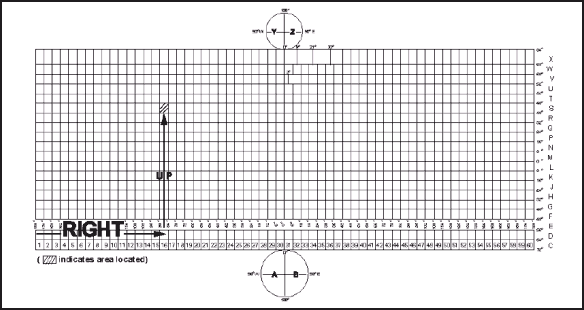Figure 4-8. UTM grid zone location

(2)   This inconvenience is eliminated by assigning “false values” to the base lines, resulting in positive values for all points within each zone. Distances are always measured RIGHT and UP (east and north as the reader faces the map), and the assigned values are called “false easting” and “false northing. “ (Figure 4-9). The false eating value for each central meridian is 500,000 meters, and the false northing value for the equator is 0 meters when measuring in the northern hemisphere and 10,000,000 meters when measuring in the southern hemisphere. The use of the UTM grid for point designation will be discussed in detail in paragraph 4-4.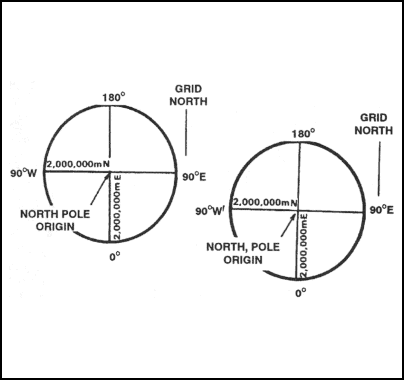Figure 4-9. False eastings and northings for the UPS grid.

b. Universal Polar Stereographic Grid. The UPS grid is used to represent the polar regions. (Figure 4-10)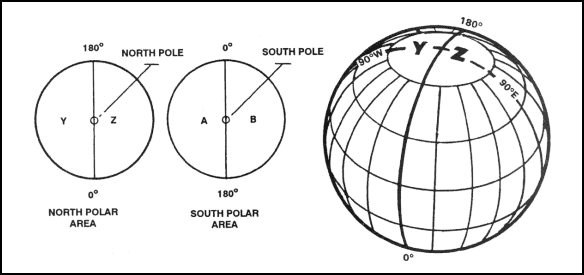Figure 4-10. Grid zone designation for UPS grid

(1) North Polar Area. The origin of the UPS grid applied to the north polar area is the north pole. The “north-south” base line is the line formed by the 0-degree and 180-degree meridians; the “east-west” base line is formed by the two 90-degree meridians.

(2) South Polar Area. The origin of the UPS grid in the south polar area is the south pole. The base lines are similar to those of the north polar area.

### 4-4. UNITED STATES ARMY MILITARY GRID REFERENCE SYSTEM

This grid reference system is designated for use with the UTM and UPS grids. The coordinate value of points in these grids could contain as many as 15 digits if numerals alone were used. The US military grid reference system reduces the length of written coordinates by substituting single letters for several numbers. Using the UTM and the UPS grids, it is possible for the location of a point (identified by numbers alone) to be in many different places on the surface of the earth. With the use of the military grid reference system, there is no possibility of this happening.

a. Grid Zone Designation. The world is divided into 60 grid zones, which are large, regularly shaped geographic areas, each of which is given a unique identification called the grid zone designation.

(1) UTM Grid. The first major breakdown is the division of each zone into areas 6° wide by 8° high and 6° wide by 12° high. Remember, for the transverse Mercator projection, the earth’s surface between 80° S and 84° N is divided into 60 N-S zones, each 6° wide. These zones are numbered from west to east, 1 through 60, starting at the 180° meridian. This surface is divided into 20 east-west rows in which 19 are 8° high and 1 row at the extreme north is 12° high. These rows are then lettered, from south to north, C through X (I and O were omitted). Any 6° by 8° zone or 6° by 12° zone is identified by giving the number and letter of the grid zone and row in which it lies. These are read RIGHT and UP so the number is always written before the letter. This combination of zone number and row letter constitutes the grid zone designation. Columbus lies in zone 16 and row S, or in grid zone designation 16S (Figure 4-8).

(2) UPS Grid. The remaining letters of the alphabet, A, B, Y, and Z, are used for the UPS grids. Each polar area is divided into two zones separated by the 0-180° meridian. In the south polar area, the letter A is the grid zone designation for the area west of the 0-180° meridian, and B for the area to the east. In the north polar area, Y is the grid zone designation for the western area and Z for the eastern area (Figure 4-10).

b. 100,000-Meter Square. Between 84° N and 80° S, each 6° by 8° or 6° by 12° zone is covered by 100,000-meter squares that are identified by the combination of two alphabetical letters. This identification is unique within the area covered by the grid zone designation. The first letter is the column designation; the second letter is the row designation (Figure 4-11). The north and south polar areas are also divided into 100,000-meter squares by columns and rows. A detailed discussion of the polar system can be found in Technical Report 8358. 1. The 100,000-meter square identification letters are located in the grid reference box in the lower margin of the map.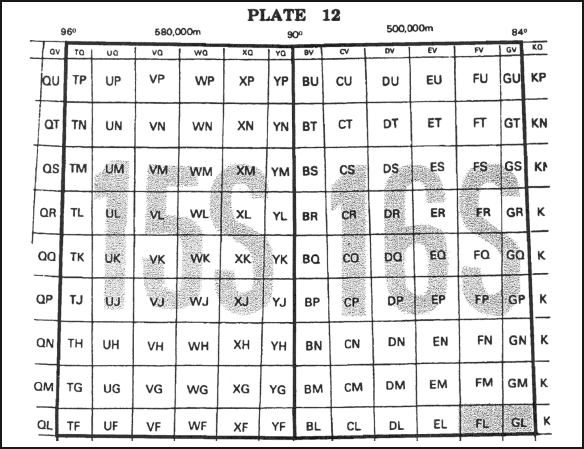Figure 4-11. Grid zone designation and 100,000-meter square identification.

c. Grid Coordinates. We have now divided the earth’s surface into 6° by 8° quadrangles, and covered these with 100,000-meter squares. The military grid reference of a point consists of the numbers and letters indicating in which of these areas the point lies, plus the coordinates locating the point to the desired position within the 100,000-meter square. The next step is to tie in the coordinates of the point with the larger areas. To do this, you must understand the following.

(1) Grid Lines. The regularly spaced lines that make the UTM and the UPS grid on any large-scale maps are divisions of the 100,000-meter square; the lines are spaced at 10,000- or 1,000-meter intervals (Figure 4-12). Each of these lines is labeled at both ends of the map with its false easting or false northing value, showing its relation to the origin of the zone. Two digits of the values are printed in large type, and these same two digits appear at intervals along the grid lines on the face of the map. These are called the principal digits, and represent the 10,000 and 1,000 digits of the grid value. They are of major importance to the map reader because they are the numbers he will use most often for referencing points. The smaller digits complete the UTM grid designation.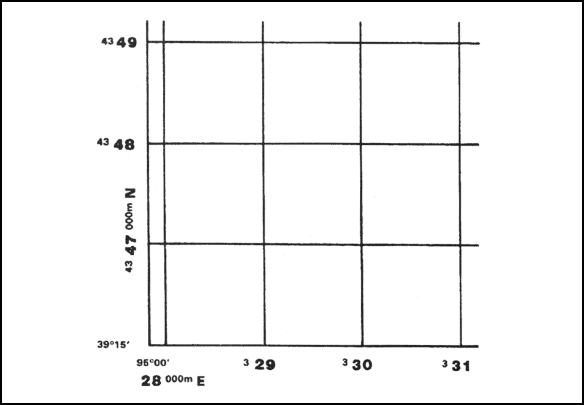Figure 4-12. Grid lines.

EXAMPLE: The first grid line north of the south-west corner of the Columbus map is labeled 3570000m N. This means its false northing (distance north of the equator) is 3,570,000 meters. The principal digits, 70, identify the line for referencing points in the northerly direction. The smaller digits, 35, are part of the false coordinates and are rarely used. The last three digits, 000, of the value are omitted. Therefore, the first grid line east of the south-west corner is labeled 689000m E. The principal digits, 89, identify the line for referencing points in the easterly direction (Figure 4-13).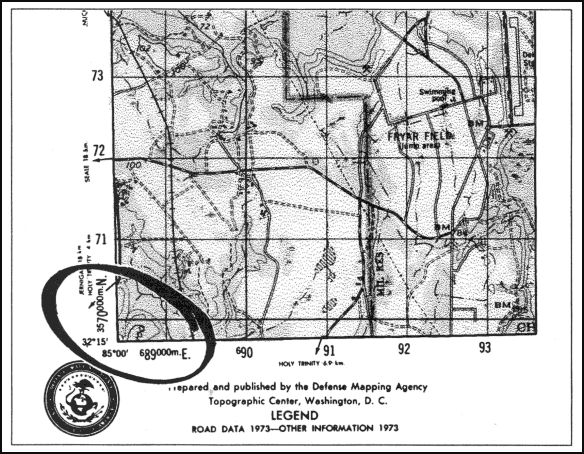Figure 4-13. Columbus map, southwest corner.

(2) Grid Squares. The north-south and east-west grid lines intersect at 90° , forming grid squares. Normally, the size of one of these grid squares on large-scale maps is 1,000 meters (1 kilometer).

(3) Grid Coordinate Scales. The primary tool for plotting grid coordinates is the grid coordinate scale. The grid coordinate scale divides the grid square more accurately than can be done by estimation, and the results are more consistent. When used correctly, it presents less chance for making errors. GTA 5-2-12, 1981, contains four types of coordinate scales (Figure 4-14).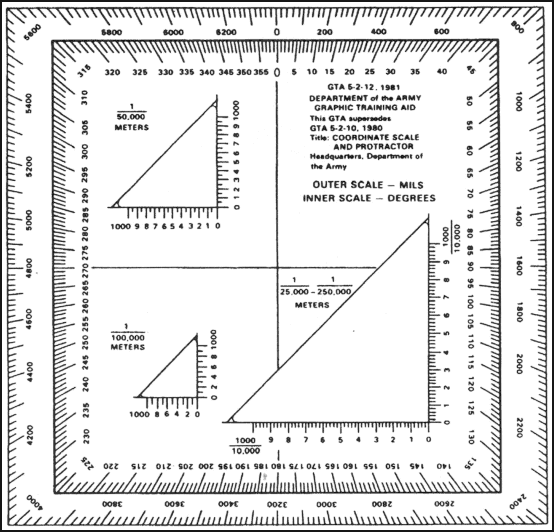Figure 4-14. Coordinate scales.

(a)   The 1:25,000/1:250,000 (lower right in figure) can be used in two different scale maps, 1:25,000 or 1:250,000. The 1:25,000 scale subdivides the 1,000-meter grid block into 10 major subdivisions, each equal to 100 meters. Each 100-meter block has five graduations, each equal to 20 meters. Points falling between the two graduations can be read accurately by the use of estimation. These values are the fourth and eighth digits of the coordinates. Likewise, the 1:250,000 scale is subdivided in 10 major subdivisions, each equal to 1,000 meters. Each 1,000-meter block has five graduations, each equal to 200 meters. Points falling between two graduations can be read approximately by the use of estimation.

(b)   The 1:50,000 scale (upper left in Figure 4-14) subdivides the 1,000-meter block into 10 major subdivisions, each equal to 100 meters. Each 100-meter block is then divided in half. Points falling between the graduations must be estimated to the nearest 10 meters for the fourth and eighth digits of the coordinates.

(c)   The 1:100,000 scale (lower left in Figure 4-14) subdivides the 1,000-meter grid block into five major subdivisions of 200 meters each. Each 200-meter block is then divided in half at 100-meter intervals.

### 4-5. LOCATE A POINT USING GRID COORDINATES

Based on the military principle for reading maps (RIGHT and UP), locations on the map can be determined by grid coordinates. The number of digits represents the degree of precision to which a point has been located and measured on a map— the more digits the more precise the measurement.

a. Without a Coordinate Scale. Determine grids without a coordinate scale by referring to the north-south grid lines numbered at the bottom margin of any map. Then read RIGHT to the north-south grid line that precedes the desired point (this first set of two digits is the RIGHT reading). Then by referring to the east-west grid lines numbered at either side of the map, move UP to the east-west grid line that precedes the desired point (these two digits are the UP reading). Coordinate 1484 locate the 1,000-meter grid square in which point X is located; the next square to the right would be 1584; the next square up would be 1485, and so forth (Figure 4-15). Locate the point to the nearest 100 meters using estimation. Mentally divide the grid square in tenths, estimate the distance from the grid line to the point in the same order (RIGHT and UP). Give complete coordinate RIGHT, then complete coordinate UP. Point X is about two-tenths or 200 meters to the RIGHT into the grid square and about seven-tenths or 700 meters UP.

RESULTS: The coordinates to the nearest 100 meters are 142847.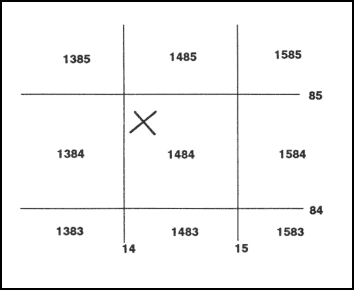Figure 4-15. Determining grids without coordinate point.

b. With a Coordinate Scale (1:25,000). In order to use the coordinate scale for determining grid coordinates, ensure that the appropriate scale is being used on the corresponding map, and that the scale is right side up. To ensure the scale is correctly aligned, place it with the zero-zero point at the lower left corner of the grid square. Keeping the horizontal line of the scale directly on top of the east-west grid line, slide it to the right until the vertical line of the scale touches the point for which the coordinates are desired (Figure 4-16). When reading coordinates, examine the two sides of the coordinate scale to ensure that the horizontal line of the scale is aligned with the east-west grid line, and the vertical line of the scale is parallel with the north-south grid line. Use the scale when precision of more than 100 meters is required. To locate the point to the nearest 10 meters, measure the hundredths of a grid square RIGHT and UP from the grid lines to the point. Point X is about 17 hundredths or 170 meters RIGHT and 84 hundredths or 840 meters UP. The coordinates to the nearest 10 meters are 14178484.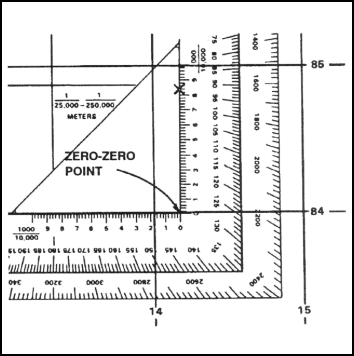Figure 4-16. Placing a coordinate scale on a grid.

NOTE:

Figure 4-17, the desired point is reported as 14818407.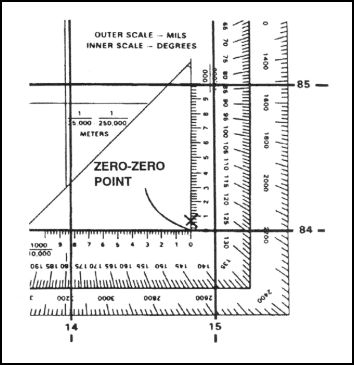Figure 4-17. Zero-zero point.

c. 1:50,000 Coordinating Scale. On the 1:50,000 coordinate scale, there are two sides: vertical and horizontal. These sides are 1,000 meters in length. The point at which the sides meet is the zero-zero point. Each side is divided into 10 equal 100-meter segments by a long tick mark and number. Each 100-meter segment is subdivided into 50-meter segments by a short tick mark (Figure 4-18). By using interpolation, mentally divide each 50-meter segment into tenths. For example, a point that lies after a whole number but before a short tick mark is identified as 10, 20, 30, or 40 meters and any point that lies after the short tick mark but before the whole number is identified as 60, 70, 80, or 90 meters.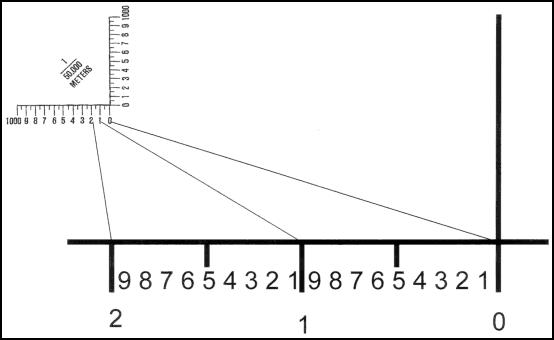Figure 4-18. 1:50,000 coordinating scale.

d. Example of Obtaining an Eight-Digit Coordinate Using 1:50,000 Scale. To ensure the scale is correctly aligned, place it with the zero-zero point at the lower left corner of the grid square. Keeping the horizontal line of the scale directly on top of the east-west grid line, slide the scale to the right until the vertical line of the scale touches the point for which the coordinates are desired (Figure 4-19). Reading right, you can see that the point lies 530 meters to the right into the grid square, which gives a right reading of 7853. Reading up, you can see that the point lies 320 meters up into the grid square, giving an up reading of 0032.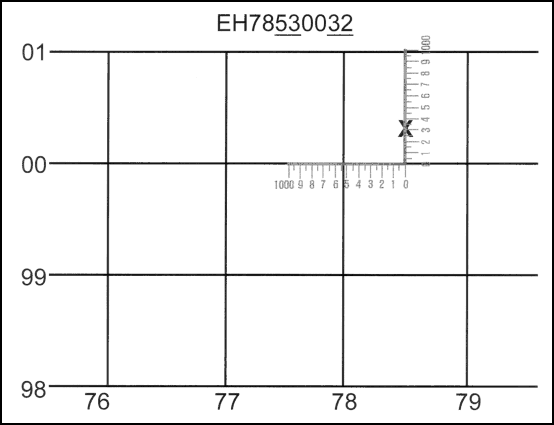Figure 4-19. Example of obtaining an eight-digit coordinate using 1:50,000 scale.

e. Recording and Reporting Grid Coordinates. Coordinates are written as one continuous number without spaces, parentheses, dashes, or decimal points; they must always contain an even number of digits. Therefore, whoever is to use the written coordinates must know where to make the split between the RIGHT and UP readings. It is a military requirement that the 100,000-meter square identification letters be included in any point designation. Normally, grid coordinates are determined to the nearest 100 meters (six digits) for reporting locations. With practice, this can be done without using plotting scales. The location of targets and other point locations for fire support are determined to the nearest 10 meters (eight digits).

NOTE:

### 4-6. LOCATE A POINT USING THE US ARMY MILITARY GRID REFERENCE SYSTEM

There is only one rule to remember when reading or reporting grid coordinates— always read to the RIGHT and then UP. The first half of the reported set of coordinate digits represents the left-to-right (easting) grid label, and the second half represents the label as read from the bottom to top (northing). The grid coordinates may represent the location to the nearest 10-, 100-, or 1,000-meter increment.

a. Grid Zone. The number 16 locates a point within zone 16, which is an area 6° wide and extends between 80° S latitude and 84° N latitude (Figure 4-8).

b. Grid Zone Designation. The number and letter combination, 16S, further locates a point within the grid zone designation 16S, which is a quadrangle 6° wide by 8° high. There are 19 of these quads in zone 16. Quad X, which is located between 72° N and 84° N latitude, is 12° high (Figure 4-8).

c. 100,000-Meter Square Identification. The addition of two more letters locates a point within the 100,000-meter grid square. Thus 16SGL (Figure 4-11) locates the point within the 100,000-meter square GL in the grid zone designation 16S. For information on the lettering system of 100,000-meter squares, see TM 5-241-1.

d. 10,000-Meter Square. The breakdown of the US Army military grid reference system continues as each side of the 100,000-meter square is divided into 10 equal parts. This division produces lines that are 10,000 meters apart. Thus the coordinates 16SGL08 would locate a point as shown in Figure 4-20. The 10,000-meter grid lines appear as index (heavier) grid lines on maps at 1:100,000 and larger.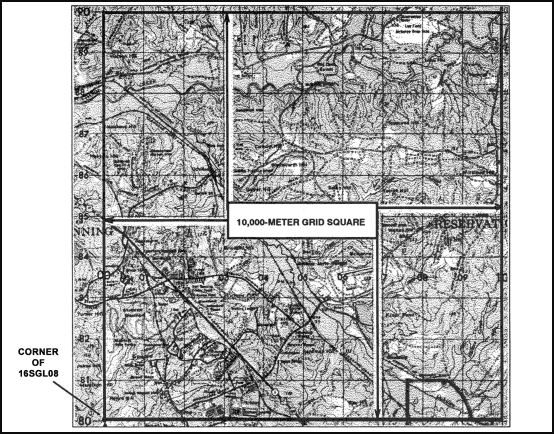Figure 4-20. The 10,000-meter grid square.

e. 1,000-Meter Square. To obtain 1,000-meter squares, each side of the 10,000-meter square is divided into 10 equal parts. This division appears on large-scale maps as the actual grid lines; they are 1,000 meters apart. On the Columbus map, using coordinates 16SGL0182, the easting 01 and the northing 82 gives the location of the southwest corner of grid square 0182 or to the nearest 1,000 meters of a point on the map (Figure 4-21).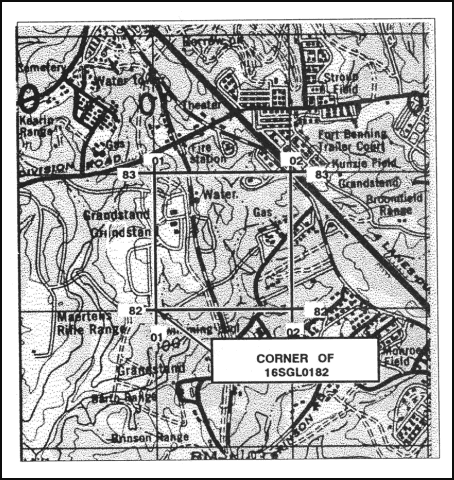Figure 4-21. The 1,000-meter grid square.

f. 100-Meter Identification. To locate to the nearest 100 meters, the grid coordinate scale can be used to divide the 1,000-meter grid squares into 10 equal parts (Figure 4-22).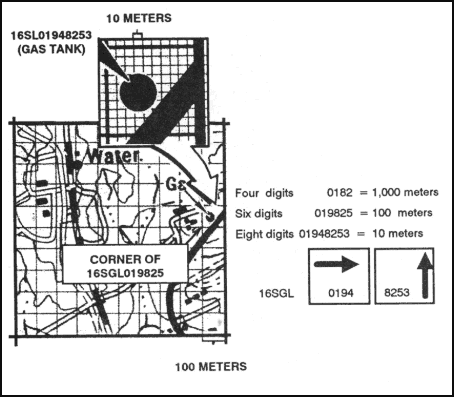Figure 4-22. The 100-meter and 10-meter grid squares.

g. 10-Meter Identification. The grid coordinate scale has divisions every 50 meters on the 1:50,000 scale and every 20 meters on the 1:25,000 scale. These can be used to estimate to the nearest 10 meters and give the location of one point on the earth’s surface to the nearest 10 meters**.**

EXAMPLE: 16SGL01948253 (gas tank) (Figure 4-22).

h. Precision. The precision of a point’s location is shown by the number of digits in the coordinates; the more digits, the more precise the location (Figure 4-22, insert).

### 4-7. GRID REFERENCE BOX

A grid reference box (Figure 4-23) appears in the marginal information of each map sheet. It contains step-by-step instructions for using the grid and the US Army military grid reference system. The grid reference box is divided into two parts.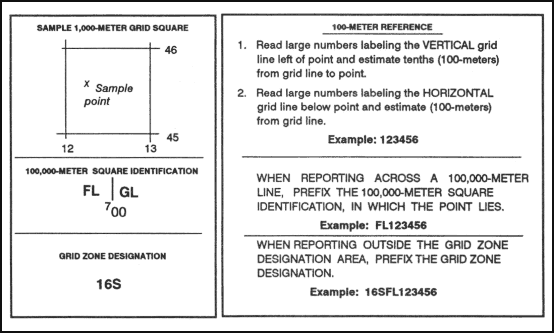Figure 4-23. Grid reference box

a.   The left portion identifies the grid zone designation and the 100,000-meter square. If the sheet falls in more than one 100,000-meter square, the grid lines that separate the squares are shown in the diagram and the letters identifying the 100,000-meter squares are given.

EXAMPLE: On the Columbus map sheet, the vertical line labeled 00 is the grid line that separates the two 100,000-meter squares, FL and GL. The left portion also shows a sample for the 1,000-meter square with its respective labeled grid coordinate numbers and a sample point within the 1,000-meter square.

b.   The right portion of the grid reference box explains how to use the grid and is keyed on the sample 1,000-meter square of the left side. The following is an example of the military grid reference:

EXAMPLE: 16S locates the 6° by 8° area (grid zone designation).

### 4-8. OTHER GRID SYSTEMS

The military grid reference system is not universally used. Soldiers must be prepared to interpret and use other grid systems, depending on the area of operations or the personnel the soldiers are operating with.

a. British Grids. In a few areas of the world, British grids are still shown on military maps. However, the British grid systems are being phased out. Eventually all military mapping will be converted to the UTM grid.

b. World Geographic Reference System (GEOREF). This system is a worldwide position reference system used primarily by the US Air Force. It may be used with any map or chart that has latitude and longitude printed on it. Instructions for using GEOREF data are printed in blue and are found in the margin of aeronautical charts (Figure 4-24). This system is based upon a division of the earth’s surface into quadrangles of latitude and longitude having a systematic identification code. It is a method of expressing latitude and longitude in a form suitable for rapid reporting and plotting. Figure 4-24 illustrates a sample grid reference box using GEOREF. The GEOREF system uses an identification code that has three main divisions.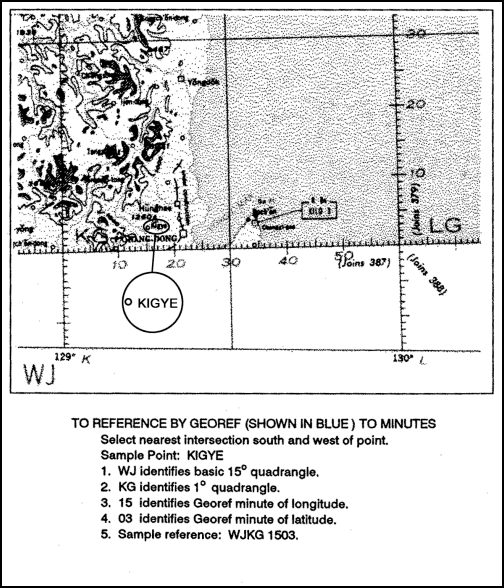Figure 4-24. Sample reference using GEOREF.

(1) First Division. There are 24 north-south (longitudinal) zones, each 15-degree wide. These zones, starting at 180 degrees and progressing eastward, are lettered A through Z (omitting I and O). The first letter of any GEOREF coordinate identifies the north-south zone in which the point is located. There are 12 east-west (latitudinal) bands, each 15-degree wide. These bands are lettered A through M (omitting I) northward from the south pole. The second letter of any GEOREF coordinate identifies the east-west band in which the point is located. The zones and bands divide the earth’s surface into 288 quadrangles, each identified by two letters.

(2) Second Division. Each 15-degree quadrangle is further divided into 225 quadrangles of 1 degree each (15 degrees by 15 degrees). This division is effected by dividing a basic 15-degree quadrangle into 15 north-south zones and 15 east-west bands. The north-south zones are lettered A through Q (omitting I and O) from west to east. The third letter of any GEOREF coordinate identifies the 1 degree north-south zone within a 15-degree quadrangle. The east-west bands are lettered A through Q (I and O omitted) from south to north. The fourth letter of a GEOREF coordinate identifies the 1 degree east-west band within a 15-degree quadrangle. Four letters identify any 1-degree quadrangle in the world.

(3) Third Division. Each of the 1-degree quadrangles is divided into 3,600 one-minute quadrangles. These one-minute quadrangles are formed by dividing the 1-degree quadrangles into 60 one-minute north-south zones numbered 0 through 59 from west to east, and 60 east-west bands numbered 0 to 59 from south to north. To designate any one of the 3,600 one-minute quadrangles requires four letters and four numbers. The rule READ RIGHT AND UP is always followed. Numbers 1 through 9 are written as 01, 02, and so forth. Each of the 1-minute quadrangles may be further divided into 10 smaller divisions both north-south and east-west, permitting the identification of 0. 1-minute quadrangles. The GEOREF coordinate for any 0. 1-minute quadrangle consists of four letters and six numbers.

### 4-9. PROTECTION OF MAP COORDINATES AND LOCATIONS

A disadvantage of any standard system of location is that the enemy, if he intercepts one of our messages using the system, can interpret the message and find our location. It is possible and can be eliminated by using an authorized low-level numerical code to express locations. Army Regulation 380-40 outlines the procedures for obtaining authorized codes.

a.   The authorized numerical code provides a capability for encrypting map references and other numerical information that requires short-term security protection when, for operational reasons, the remainder of the message is transmitted in plain language. The system is published in easy-to-use booklets with sufficient material in each for one month’s operation. Sample training editions of this type of system are available through the unit’s communications and electronics officer.

b.   The use of any encryption methods other than authorized codes is, by regulation, unauthorized and shall not be used.